# How to☝️ Extract a Substring in Google Sheets: The Ultimate Guide

To extract a substring in Google Sheets, click on a cell, go to the Formula bar, and enter one of the following formulas: =LEFT(A1, 4), =MID(A1, 6, 9), or =RIGHT(A1, 3). Lastly, press Enter to get the result.

Quick Jump

## What Is a Substring?

In Google Sheets, a substring is a contiguous sequence of characters within a string of data. For example, the string “what a wonderful day” contains the substrings “what,” “a,” “wonderful,” and “day.”

## How to Extract a Substring in Google Sheets: The Essentials

Extracting a substring from a larger string is a common operation in Google Sheets. There are several ways to do this. Let’s take a look at the various options we have available.

### Extract Substring from the Left Side of the String

To extract text from the beginning of a string, simply do the following:

1. Click on a blank cell where you want the result.
2. Navigate to the Formula bar and enter the formula =LEFT(A1, 4), where A1 is the cell with the text and 4 is the number of letters you need to copy into the new cell.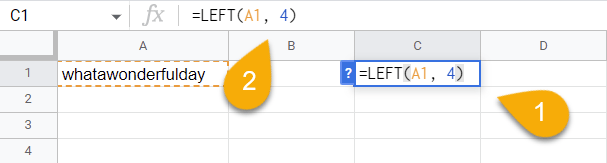1. Press the Enter key on your keyboard.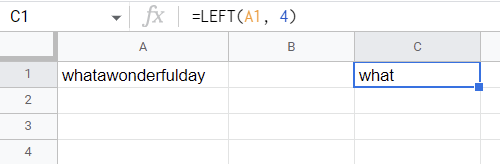Super easy!

### Extract Substring from the Middle of the String

The following steps will show you how to extract text from the middle of a string. Let’s see how it works!

1. Select a blank cell.
2. In the Formula bar, type the formula =MID(A1, 6, 9). Here A1 is the cell with the text, 6 is the letter from which the count begins, and 9 is the number of letters you want to extract.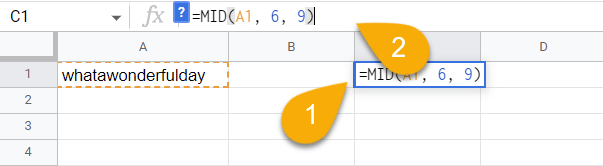3.  Hit the Enter key.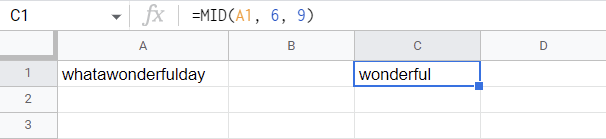Easy as ABC!

### Extract Substring from the Right Side of the String

Next, let’s consider how to extract text from the end of a string!

1. Select a blank cell where you want the result to show.
2. Go to the Formula bar and enter the formula =RIGHT(A1, 3), where A1 is the cell with the text, and 3 is the number of letters you need to extract.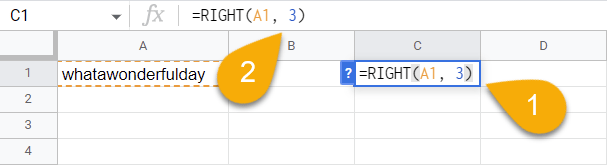3. Press Enter.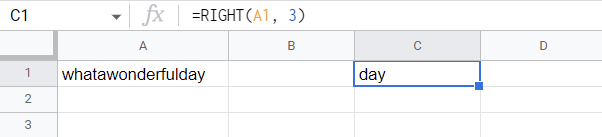And there you have it!

## How to Extract a Substring Using Certain Text

Learn how to extract a substring using specific text by following the formulas in this section.

### Extract a Substring Before a Certain Text

If you want to extract from the beginning of a string based on a specific word, there is a way to do this. Take a look below:

1. Select the cell where you want the result.
2. In the Formula bar, enter the formula =LEFT(A1, SEARCH(“green”, A1)-1). A1 is the cell with text, and green is the word from which counting begins.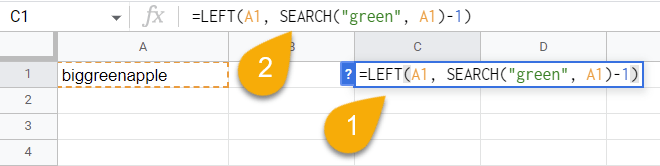3. Press the Enter key on your keyboard.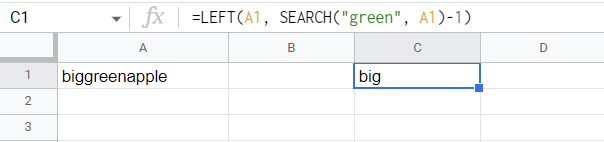That’s all it takes! Here is the result.

### Extract a Substring After a Certain Text or Character

Check out the following step-by-step guide to extract a substring after a specific text or character:

1. Click on a blank cell.
2. Go to the Formula bar and type the formula =REGEXREPLACE(A1,”(.*)green(.*)”,”\$2″), where A1 contains the text and green is the word from which counting begins.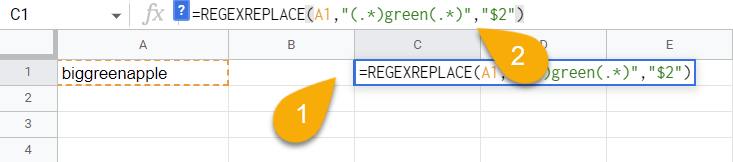3. Press the Enter key.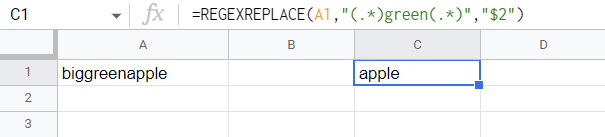Voila!

## How to Pull Text from Between Two Occurrences of a Character

If you need to find and extract the text from between two special characters, you can use the method below to find what you need:

1. Click on a blank cell where you want the result.
2. Navigate to the Formula bar and use the formula =MID(A1, SEARCH(“_”,A1) + 1, SEARCH(“_”,A1,SEARCH(“_”,A1)+1) – SEARCH(“_”,A1) – 1). A1 is the cell with your text, and “_ are the characters between which the desired word is located.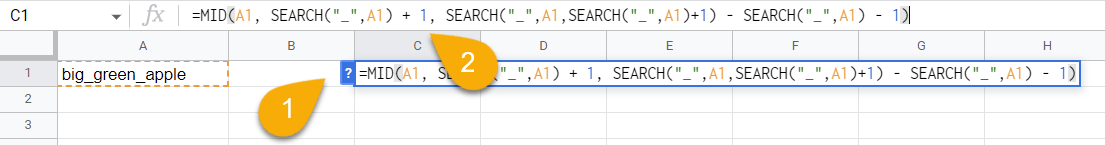3. Hit the Enter key.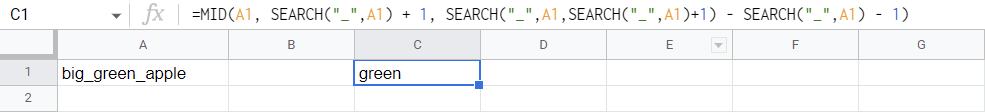Here is the desired result!

## How to Extract the Nth Word from a Text String

Take a look at the process below to see how to extract the nth word from a text string:

1. Select a blank cell.
2. Go to the Formula bar and enter the formula =TRIM(MID(SUBSTITUTE(\$A\$1,” “,REPT(” “,LEN(\$A\$1))), (B1-1)*LEN(\$A\$1)+1, LEN(\$A\$1))). Here A1 contains the text and B1 is the cell with the word number you need to pull (in this case, 3, or the 3rd number).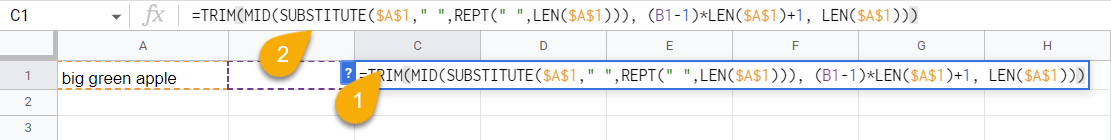3. Tap Enter.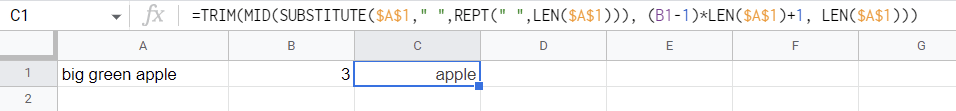And there is the result!

## How to Extract a Substring Between Parentheses

Check out this guide on how to pull text from between parentheses in a string:

1. Select the cell where you want the result.
2. In the Formula bar, enter the formula =MID(A1,SEARCH(“(“,A1)+1, SEARCH(“)”,A1)-SEARCH(“(“,A1)-1). Here, A1 is the text-filled cell.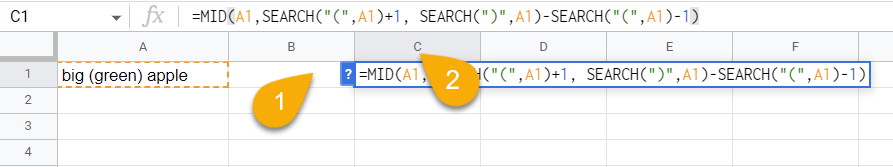3. Lastly, hit the Enter key.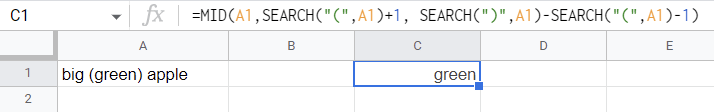Great! We have extracted the word between the parentheses.

## How to Replace One Substring With Another

If you need to replace one substring with another, you can do it in just a few seconds:

1. Click on a blank cell.
2. Move to the Formula bar and type the formula =REPLACE(A1, FIND(“132”, A1), 3, “123”), where A1 contains text, and 132 is the text you need to replace with 123.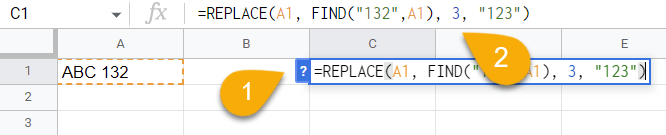3.  Finally, press Enter.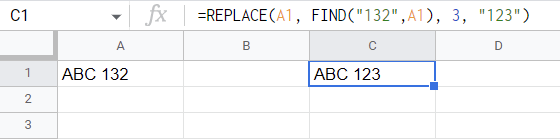Piece of cake!

## How to Extract a Number from a String

Here are some tips that will help you extract a number from the string in case you need to do so:

### Extract Number Substring from the Left of the String

It is recommended to use the formula below if the numbers are at the beginning of the cell.

1. Choose the cell where you need the extracted number to go.
2. In the Formula bar, type the formula =REGEXEXTRACT(A1,”^\d+”). A1 is the cell with the data.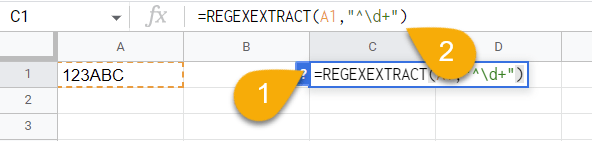3. Press the Enter key.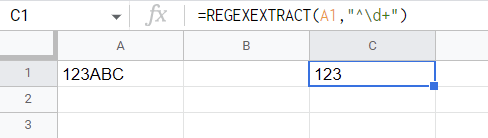Easy!

### Extract Number Substring from the Right of the String

Now we will show how to extract numbers if they are located at the end of the entire text:

1. Select the cell where you want the extracted number to go.
2. Move to the Formula bar and enter the formula =REGEXEXTRACT(A1,”\d+”). Here A1 contains the text.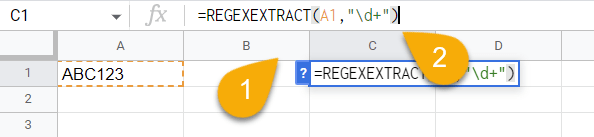3. Hit Enter.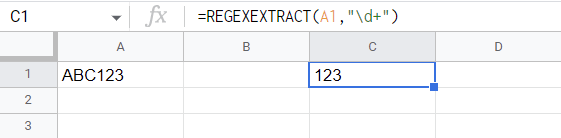There you go! You have done it!

### Extract Substring from Anywhere in a String

If there are numbers in the middle of your text, you can use the following formula to extract them into a separate cell. Let’s take a look:

1. Click on a blank cell.
2. Go to the Formula bar and enter the formula =REGEXEXTRACT(A1, “\d+”), where A1 contains your text.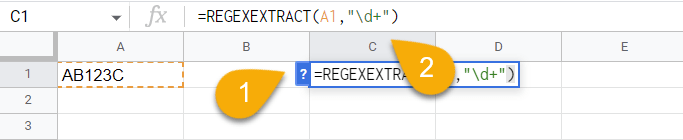3. Press Enter.The process is super simple!